## On the History of Proving False ‘Theorems’

Despite its inhumanly pristine appearance in textbooks and the classroom, mathematics is a human endeavour, sometimes stimulated by debate and controversy.

We typically view mathematical theorems as necessarily true once proven; that is, they cannot be demonstrated false, provided a ‘good’ proof has been supplied (one that is valid and has true premises). Furthermore, the methods of proving such theorems are usually viewed as pristine and untainted by any empirical considerations or scientific methodology, involving only deep reflection about mathematical facts. I would like to suggest that this default characterisation is mistaken, and that mathematical practice at least sometimes takes on a methodology akin to that of the natural sciences. In this sense, mathematical progress is made via bold conjectures followed by attempts to find falsifications of them. Now, this is not at all apparent, since one’s usual exposure to mathematical practice is in the classroom, where one is presented with theorems with the apparent aura of necessary truth that appear simply to be the product of some great feat of armchair reflection by a mathematician.

Consider Euler’s Formula: F – E + V = 2. This theorem claims that all polyhedra satisfy this formula, where F is the number of faces, E is the number of edges (or sides of the faces) and V is the number of vertices (or corners) of any polyhedron. When I was first presented with this theorem, it was claimed that the idea originated with Euler (which is true), but in addition, that he demonstrated by valid proof that it is true (which is false). In actual fact, Euler’s Formula was only demonstrated true approximately two centuries after his initial proof. The historical dispute about this theorem will be instrumental in my aim to display how mathematics can take on a more scientific flavour of conjecture and refutation. So, let us turn to this.

Euler began with the following question: Is there a universal way one can characterise polyhedra, within 3-D geometry, in an analogous manner to the trivial characterisation that all polygons share (simply V = E), within 2-D geometry?[i] In 1758 he provided a proof, but for simplicity I will briefly sketch Cauchy’s ingenious proof from 1813[ii]:

To demonstrate: For any polyhedron (we take a cube as an example), once it is projected on a 2-D plane it will satisfy F – E + V = 1.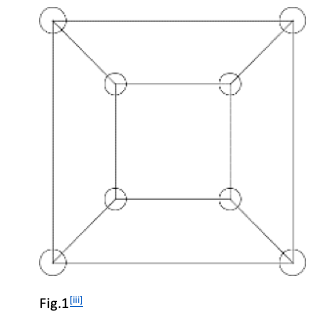1. For any polyhedron, remove one of its faces and project the remaining surfaces on the 2-D plane (Fig. 1). Since only one face was removed, we obtain a polygon satisfying F – E + V = 1 as the reader can check.

2. Triangulate the polygon: this is simply when a polygon is sub-divided into triangles meeting edge-to-edge with same number of vertices contained in the pre-triangulated polygon (Fig. 2). The triangulation increases both E and F by same amount, so F – E + V = 1.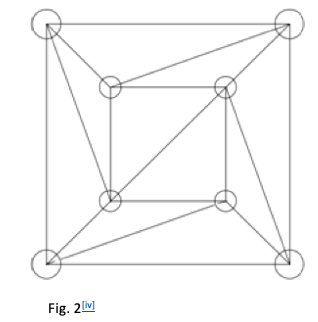3. Beginning with those on the exterior, remove triangles one by one. There are two possibilities when removing a triangle: either one edge and one face will be removed, or one face, one vertex and two edges will be removed (Fig. 3 & 4). Consequently, this preserves F – E + V =1.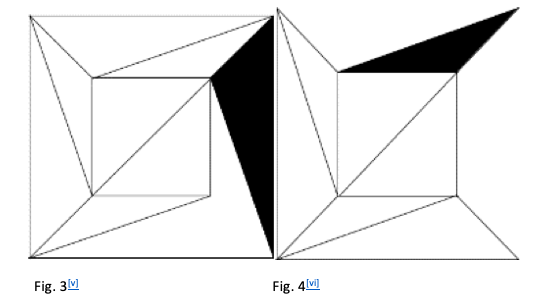Repeating this process until we reach the last triangle, we note that this triangle trivially satisfies F – E + V = 1. Therefore, for any polyhedron, once it is projected onto the 2-D plane it will satisfy F – E + V = 1. And so for the original polyhedron, which was just the same as the polygon except that it had one more face (which we removed in the first step), F – E + V = 1 + 1 = 2. Q.E.D.

Did Cauchy’s proof demonstrate the truth of Euler’s Formula? There are many issues with this proof, but there is an essential concern: does the projection in the first step hold for all polyhedra? And how do we know that this is true? If it is true, we need an additional proof for its truth – a lemma. Nonetheless, this fact did not perturb mathematicians such as Crelle,[vii] Matthiessen,[viii]  Jonquières[ix] to mention a few. They thought Cauchy’s proof demonstrated the irrevocable truth of Euler’s Formula.

Yet, a little less than a year after Cauchy’s proof, L’Huilier claimed he had a counterexample.[x] It involves “a solid bounded by a pair of nested cubes” where one cube is inside the other, but neither cube touches the other (Fig. 5).[xi] This polyhedron falsifies the possibility of a proof for the lemma, since if a face is removed from the inner cube, then the polyhedron is not projectable onto a 2-D plane (likewise if we remove the face of the outer cube). Furthermore, Euler’s formula is false for this polyhedron; it only satisfies the formula F – E + V = 4 (check it below). Furthermore, Euler’s formula is false for this polyhedron; it only satisfies the formula F – E + V = 4 (check it below).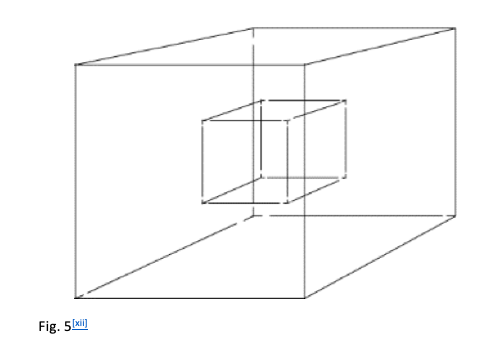Now, this is an outright falsification, and so the theorem should be rejected. But this is where the methodology of mathematics starts to resemble that of science. In science, when confronted with a falsification of a hypothesis, there is an attempt to explain the falsification away in a non-ad hoc manner. This is done by modifying the hypothesis; and this is exactly what happened in the historical development of Euler’s Formula. In response to such counterexamples, mathematicians changed the definition of a polyhedron. We have been playing fast and loose with the definition of a polyhedron, so I will define it now as Euler inter alia[xiii] defined it: “A polyhedron is a solid whose surface consists of polygonal faces”.[xiv] After L’Huilier’s counterexample, the mathematical community changed their definition to that of: “A polyhedron is a surface consisting of a system of polygons”.[xv],[xvi] This definition precludes the counterexample above from being characterised as a polyhedron; it is really “two polyhedra – two surfaces, one completely inside the other”.[xvii]

But with this definition accepted, it gave rise to another counterexample by Hessel 1832.[xviii]  Consider a twin- tetrahedron; this is a polyhedron constructed when two polyhedra are connected by either an edge or vertex (see Fig. 6 & 7). For any twintetrahedron, it will only satisfy the formula: F – E + V = 3. Therefore, it proved to be another falsification of Euler’s Formula under the new definition of a polyhedron.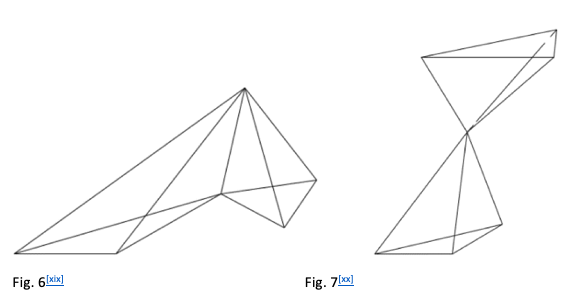What happened next? You guessed it; the definition of a polyhedron changed again! This new definition was clearly articulated by Möbius in 1865, to avoid characterising twintetrahedra as polyhedra, and was formulated roughly as: “[A] polyhedron [is] a system of polygons arranged in such a way that (1) exactly two polygons meet at every edge and (2) it is possible to get from the inside of any polygon to the inside of any other polygon by a route which never crossed any edge at a vertex.”[xxi],[xxii] I will leave it up to the reader to check, but it suffices to say that this definition avoids the first counterexample by L’Huilier and the two twintetrahedra counterexamples presented by Hessel (the first criterion excludes Fig. 6 and the second criterion excludes Fig. 7). This only brings us to 1865. But by now you would not be mistaken in predicting that a slew of counterexamples and additional refinements to the definition of a polyhedron ensued, though I will leave the examples here.[xxiii]

This brief historical excursus above should have made it apparent that a central notion to Euler’s Formula was poorly defined – the polyhedron. It was only through a laborious and iterated process of conjecture, proof, theorem, refutation by counterexample, and then amendments to the definition of the polyhedron, that mathematicians came to understand what polyhedra were; and from that, successfully characterise all polyhedra as satisfying the formula: F – E + V = 2. This should suggest that mathematical theorems and proofs are not trivial or only the product of deep reflection. It requires a community, and a messy to and fro between its members by process of proof, refutation, amendment, proof, refutation…. This is how natural sciences generally progress and, as I have been suggesting by our historical analysis, sometimes mathematics too. But this leaves us with a curious result; it potentially permits the idea that we can prove a conjecture that is false. (For example, Cauchy’s proof of Euler’s Formula in 1813; the conjecture he proved was blatantly false under his definition of the polyhedron). Does this mean that mathematicians can prove false theorems? Does it matter? It might be objected that this just displays that there was something wrong with Cauchy’s proof, so mathematicians cannot prove false theorems. Notwithstanding this curious debate, at base the discussion ought to have shown that mathematics is a human endeavour, sometimes stimulated by debate and controversy over the truth of theorems, despite its inhumanly pristine appearance in textbooks and the classroom.

MSocSc in Philosophy,
UNIVERSITY OF CAPE TOWN

This work was suggested and revised by

DR GREGORY FRIED
Department of Philosophy,
UNIVERSITY OF CAPE TOWN

[i] Euler, L.. “Elementa Doctrinae Solidorum Scientiarum Petropolitanae.” Novi Commentarii Academiae 4 (1758): 109–140.

[ii] Cauchy, A. L.. “Recherches sur les Polyèdres.” Journal de l’École Polytechnique 9 (1813): 68–86.

[iii] Lakatos, Imre. Proofs and refutations: The logic of mathematical discovery. (Cambridge: Cambridge university press, 2015): 8.

[iv] Ibid., 8.

[v] Ibid., 8.

[vi] Ibid., 8.

[vii] Crelle, A. L.. Lehrbuch der Elemente der Geometrie, vols. 1 and 2. (Berlin: Reimer, 1826–7): 668-671.

[viii] Matthiessen, L.. “Über die Scheinbaren Einschränkungen des Euler’schen Satzes von den Polyedern.” Zeitschrift für Mathematik und Physik 8 (1863): 449.

[ix] Jonquières, E. de.. “Note sur un Point Fondamental de la Théoriedes Polyèdres.” Comptes Rendus des Séances de l’Académie des Sciences 110 (1890): 110-115. & Jonquières, E. de.. “Note sur le Théorème d’Euler dans la Théorie des Polyèdres.” Comptes Rendus des Séances de l’Académie des Sciences 110 (1890): 169–173.

[x] Lhuilier, S. A. J.. “Mémoire sur la Polyèdrométrie.” Annales de Mathématiques, Pures et Appliquées 3 (1812–13): 194.

[xi] Lakatos, Imre. Proofs and refutations: The logic of mathematical discovery. (Cambridge: Cambridge university press, 2015): 13

[xii] Ibid., 13.

[xiii] Euler, L.. “Elementa Doctrinae Solidorum Scientiarum Petropolitanae.” Novi Commentarii Academiae 4 (1758): 109–140. & Legendre, A.-M.. Éléments de Géométrie. Eighth edition. (Paris: Didot, 1809).

[xiv] Lakatos, Imre. Proofs and refutations: The logic of mathematical discovery. (Cambridge: Cambridge university press, 2015): 14.

[xv] Ibid., 14.

[xvi] This definition can be found in: Jonquières, E. de.. “Note sur le Théorème d’Euler dans la Théorie des Polyèdres.” Comptes Rendus des Séances de l’Académie des Sciences 110 (1890): 170.

[xvii] Lakatos, Imre. Proofs and refutations: The logic of mathematical discovery. (Cambridge: Cambridge university press, 2015): 14-15.

[xviii] Hessel, J. F.. “Nachtrag zu dem Euler’schen Lehrsatze von Polyedern.” Journal für die Reine und Angewandte Mathematik 8 (1832): 13.

[xix] Lakatos, Imre. Proofs and refutations: The logic of mathematical discovery. (Cambridge: Cambridge University Press, 2015): 15.

[xx] Ibid., 15.

[xxi] Ibid., 15.

[xxii] Möbius, A. F.. “Über die Bestimmung des Inhaltes eines Polyeders.” Berichte Königlich-Sächsischen Gesellschaft der Wissenschaften, Mathematisch–Physikalische Classe 17 (1865): 32.

[xxiii] For more counterexamples to Euler’s Formula and amendments to the definition of a polyhedron see: Lakatos, Imre. Proofs and refutations: The logic of mathematical discovery. (Cambridge: Cambridge University Press, 2015): 1-23.